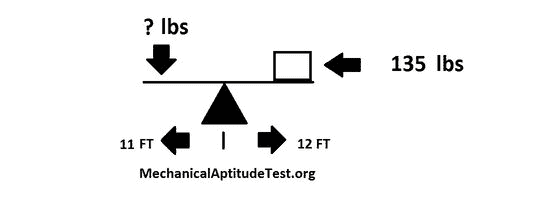# Levers

## Mechanical Aptitude Test – Levers

A lever is defined as: “A lever is a beam attached to ground using a hinge, or pivot, known as a fulcrum. The perfect lever doesn’t dissipate or store energy, meaning there isn’t any friction from the hinge or bending from the beam. In this instance, the power in to the lever equals the power out, as well as the ratio of output to input force is given by the ratio of the distances from the fulcrum to the points of application of these forces. This is what’s called the law of the lever. ”

The formula used to calculate levers is as follows:  W x D1 = F x D2

W =  Weight

D1 = Distance from fulcrum to weight

F=    Force required

D2= Distance from fulcrum to force point

Here are some examples to get your started:

Example 1:

How much force must we exert in order to lift the weight?a) 127.47

b) 147.27

c) 152.39

d) 176.56

In this example we see that the weight(W) is 135 lbs. We are aware that the distance between the weight and the fulcrum is 12(D1). The final piece of information that is given to us is that the distance between the fulcrum and the force required is 11(D2).

Therefore, by modifying the formula for what is required, you come up with the following.

F=(W x D1)/D2

Now when you insert the values you are given this.

F=(135 x 12)/11

F=(1620)/11

F= 147.27

Find more lever examples here.

[ CONTINUE TO MAPS ]